# Difference between revisions of "SPC/E model of water"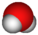This article is a 'stub' about water and/or ice. It has no, or next to no, content. It is here at the moment to help form part of the structure of SklogWiki. If you add material to this article, remove the {{Stub-water}} template from this page.
The extended simple point charge model, SPC/E is a slight reparameterisation of the SPC model of water, with a modified value for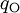$q_{\mathrm{O}}$. The molecule is modelled as a rigid isosceles triangle, having charges situated on each of the three atoms. Apart from Coulombic interactions, the molecules interact via long-range Lennard-Jones sites, situated on the oxygen atoms. The parameters are as follows:
 parameter value$\sigma$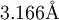$3.166 {\mathrm {\AA}}$$\epsilon$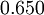$0.650$ kJ mol-1$r_\mathrm{OH}$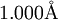$1.000\mathrm{\AA}$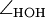$\angle_\mathrm{HOH}$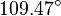$109.47^{\circ}$$q_{\mathrm{O}}$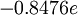$-0.8476 e$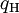$q_{\mathrm{H}}$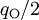$q_{\mathrm{O}}/2$ (charge neutrality)$r_\mathrm{OM}$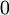$0$ (charge sits on oxygen)